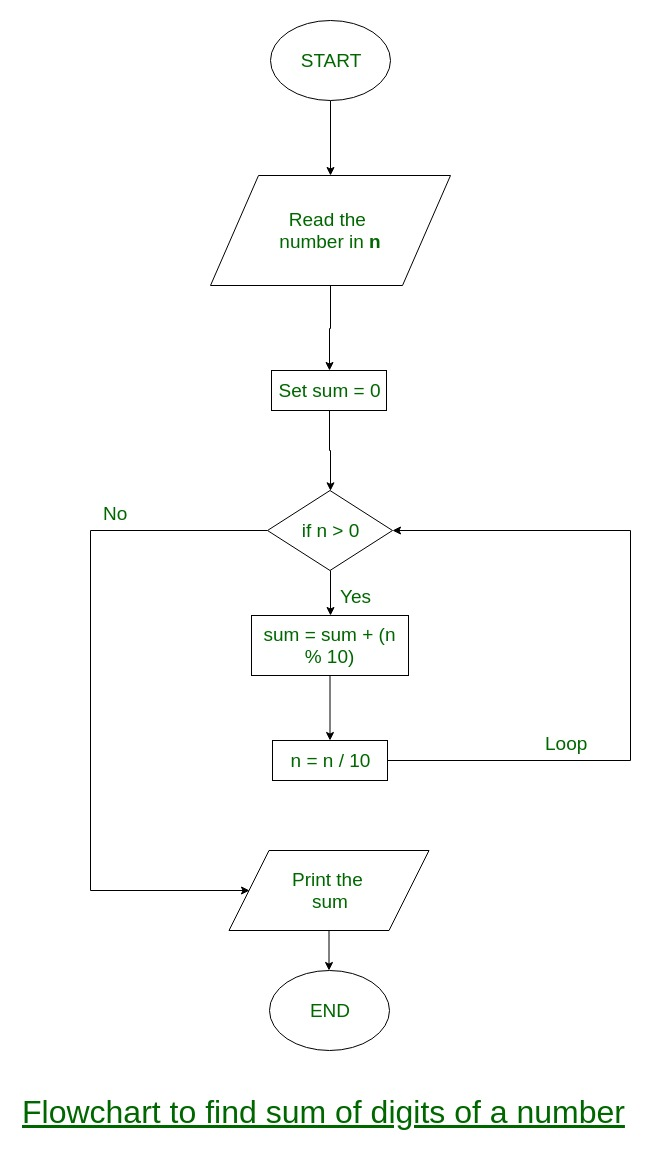Related Articles

# Check if the product of digit sum and its reverse equals the number or not

• Last Updated : 26 Apr, 2021

Given a number, check whether the product of digit sum and reverse of digit sum equals the number or not.
Examples:

```Input : 1729
Output : Yes
Explanation:
digit sum = 1 + 7 + 2 + 9
= 19
Reverse of digit sum = 91
Product = digit sum * Reverse of digit sum
= 19 * 91
= 1729

Input : 2334
Output : No```

Flowchart:Approach :
1. Find the sum of digits of a given number.
2. Reverse the digit sum output.
3. Product of digit sum and reverse of digit sum.
If the product equals to the original number then print “Yes” else print “No”.

## C++

 `// CPP implementation to check if the``// product of digit sum and its``// reverse equals the number or not``#include``using` `namespace` `std;` `// Function that returns number after``// performing operations.``int` `check(``int` `num)``{``    ``int` `digitSum = 0;``    ` `    ``// loop used to count digit sum``    ``// of numbers.``    ``while``(num > 0)``    ``{``        ``digitSum = digitSum + num % 10;``        ``num = num / 10;``    ``}``    ``int` `temp = digitSum;``    ``int` `reverseDigitSum = 0;``    ` `    ``// loop that reverse digit sum.``    ``while``(temp > 0)``    ``{``        ``int` `rem = temp % 10;``        ``reverseDigitSum = reverseDigitSum * 10``                        ``+ rem;``        ``temp = temp / 10;``    ``}``    ` `    ``// product of digit sum and reverse digit``    ``// sum and assign it to number variables.``    ``int` `number = digitSum * reverseDigitSum;``    ``return` `number;``}` `// Driver function``int` `main()``{``    ``int` `num = 1729;` `    ``// call check() function.``    ``int` `x = check(num);` `    ``// check if original number equals``    ``// to x or not``    ``if` `(num == x)``        ``cout << ``"Yes"``;``    ``else``        ``cout << ``"No"``;``    ``return` `0;``}`

## Java

 `// JAVA implementation to check if the``// product of digit sum and its``// reverse equals the number or not``class` `GFG {``     ` `    ``// Function that returns number after``    ``// performing operations.``    ``static` `int` `check(``int` `num)``    ``{``        ``int` `digitSum = ``0``;``         ` `        ``// loop used to count digit sum``        ``// of numbers.``        ``while``(num > ``0``)``        ``{``            ``digitSum = digitSum + num % ``10``;``            ``num = num / ``10``;``        ``}``        ``int` `temp = digitSum;``        ``int` `reverseDigitSum = ``0``;``         ` `        ``// loop that reverse digit sum.``        ``while``(temp > ``0``)``        ``{``            ``int` `rem = temp % ``10``;``            ``reverseDigitSum = reverseDigitSum * ``10``                            ``+ rem;``            ``temp = temp / ``10``;``        ``}``         ` `        ``// product of digit sum and reverse digit``        ``// sum and assign it to number variables.``        ``int` `number = digitSum * reverseDigitSum;``        ``return` `number;``    ``}``     ` `    ``// Driver function``    ``public` `static` `void` `main(String args[])``    ``{``        ``int` `num = ``1729``;``     ` `        ``// call check() function.``        ``int` `x = check(num);``     ` `        ``// check if original number equals``        ``// to x or not``        ``if` `(num == x)``            ``System.out.println(``"Yes"``);``        ``else``           ``System.out.println(``"No"``);``    ``}``}` `// This code is contributed by Nikita Tiwari`

## Python3

 `# Python implementation to check if the``# product of digit sum and its``# reverse equals the number or not`  `# Function that returns number after``# performing operations.``def` `check(num) :``    ``digitSum ``=` `0``    ` `    ``# loop used to count digit sum``    ``# of numbers.``    ``while``(num !``=` `0``) :``        ``digitSum ``=` `digitSum ``+` `num ``%` `10``        ``num ``=` `num ``/``/` `10``    ` `    ``temp ``=` `(``int``)(digitSum)``    ``reverseDigitSum ``=` `0``          ` `    ``# loop that reverse digit sum.``    ``while``(temp !``=` `0``) :``        ``rem ``=` `temp ``%` `10``        ``reverseDigitSum ``=` `reverseDigitSum ``*` `10` `+` `rem``        ``temp ``=` `temp ``/` `10``            ` `    ``# product of digit sum and reverse digit``    ``# sum and assign it to number variables.``    ``number ``=` `digitSum ``*` `reverseDigitSum``    ``return` `number``    ` `# Driver function``num ``=` `1729` `# call check() function.``x ``=` `(check(num))` `# check if original number ``# equals to x or not``if` `(num ``=``=` `x) :``    ``print``(``"Yes"``)``else` `:``    ``print``(``"No"``)``    ` `    ` `# This code is contributed by Nikita Tiwari.`

## C#

 `// Code to check if the product``// of digit sum and its reverse``// equals the number or not``using` `System;` `class` `GFG {` `    ``// Function that returns number``    ``// after performing operations.``    ``static` `int` `check(``int` `num)``    ``{``        ``int` `digitSum = 0;` `        ``// loop used to count digit``        ``// sum of numbers.``        ``while` `(num > 0) {``            ``digitSum = digitSum + num % 10;``            ``num = num / 10;``        ``}` `        ``int` `temp = digitSum;``        ``int` `reverseDigitSum = 0;` `        ``// loop that reverse digit sum.``        ``while` `(temp > 0) {``            ``int` `rem = temp % 10;``            ``reverseDigitSum = reverseDigitSum * 10``                              ``+ rem;``            ``temp = temp / 10;``        ``}` `        ``// product of digit sum and``        ``// reverse digit sum, assign``        ``// it to number variables.``        ``int` `number = digitSum * reverseDigitSum;``        ``return` `number;``    ``}` `    ``// Driver function``    ``public` `static` `void` `Main()``    ``{``        ``int` `num = 1729;` `        ``// call check() function.``        ``int` `x = check(num);` `        ``// check if original number``        ``// equals to x or not``        ``if` `(num == x)``            ``Console.WriteLine(``"Yes"``);``        ``else``            ``Console.WriteLine(``"No"``);``    ``}``}` `// This code is contributed by Anant Agarwal.`

## PHP

 ` 0)``    ``{``        ``\$digitSum` `= ``\$digitSum` `+``                    ``\$num` `% 10;``        ``\$num` `= (int)(``\$num` `/ 10);``    ``}``    ``\$temp` `= ``\$digitSum``;``    ``\$reverseDigitSum` `= 0;``    ` `    ``// loop that reverse``    ``// digit sum.``    ``while``(``\$temp` `> 0)``    ``{``        ``\$rem` `= ``\$temp` `% 10;``        ``\$reverseDigitSum` `= ``\$reverseDigitSum` `*``                                   ``10 + ``\$rem``;``        ``\$temp` `= (int)(``\$temp` `/ 10);``    ``}``    ` `    ``// product of digit sum``    ``// and reverse digit``    ``// sum and assign it``    ``// to number variables.``    ``\$number` `= ``\$digitSum` `* ``\$reverseDigitSum``;``    ``return` `\$number``;``}` `// Driver Code``\$num` `= 1729;` `// call check() function.``\$x` `= check(``\$num``);` `// check if original``// number equals``// to x or not``if` `(``\$num` `== ``\$x``)``echo``(``"Yes"``);``else``    ``echo``(``"No"``);` `// This code is contributed by Ajit.``?>`

## Javascript

 ``

Output:

`Yes`

This article is contributed by Dharmendra Kumar. If you like GeeksforGeeks and would like to contribute, you can also write an article using contribute.geeksforgeeks.org or mail your article to contribute@geeksforgeeks.org. See your article appearing on the GeeksforGeeks main page and help other Geeks.